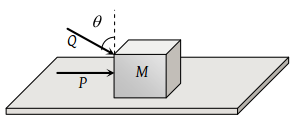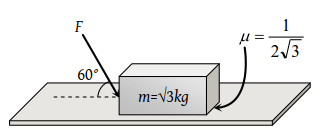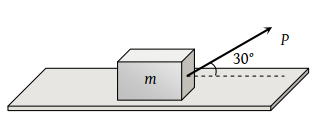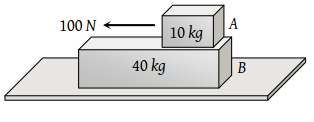A force of 750 N is applied to a block of mass 102 kg to prevent it from sliding on a plane with an inclination angle 30° with the horizontal. If the coefficients of static friction and kinetic friction between the block and the plane are 0.4 and 0.3 respectively, then the frictional force acting on the block is

(1) 750 N

(2) 500 N

(3) 345 N

(4) 250 N

Concept Questions :-

Friction
High Yielding Test Series + Question Bank - NEET 2020

Difficulty Level:

A body takes just twice the time as long to slide down a plane inclined at 30o to the horizontal as if the plane were frictionless. The coefficient of friction between the body and the plane is

(1) $\frac{\sqrt{3}}{4}$

(2) $\sqrt{3}$

(3) $\frac{4}{3}$

(4) $\frac{3}{4}$

Concept Questions :-

Friction
High Yielding Test Series + Question Bank - NEET 2020

Difficulty Level:

A body takes time t to reach the bottom of an inclined plane of angle θ with the horizontal. If the plane is made rough, time taken now is 2t. The coefficient of friction of the rough surface is

(1) $\frac{3}{4}\mathrm{tan}\theta$

(2) $\frac{2}{3}\mathrm{tan}\theta$

(3) $\frac{1}{4}\mathrm{tan}\theta$

(4) $\frac{1}{2}\mathrm{tan}\theta$

Concept Questions :-

Friction
High Yielding Test Series + Question Bank - NEET 2020

Difficulty Level:

A block is kept on an inclined plane of inclination θ of length l. The velocity of particle at the bottom of inclined is (the coefficient of friction is μ)

(1) $\sqrt{2gl\left(\mu \mathrm{cos}\theta -\mathrm{sin}\theta \right)}$

(2) $\sqrt{2gl\left(\mathrm{sin}\theta -\mu \mathrm{cos}\theta \right)}$

(3) $\sqrt{2gl\left(\mathrm{sin}\theta +\mu \mathrm{cos}\theta \right)}$

(4) $\sqrt{2gl\left(\mathrm{cos}\theta +\mu \mathrm{sin}\theta \right)}$

Concept Questions :-

Friction
High Yielding Test Series + Question Bank - NEET 2020

Difficulty Level:

A block of mass m lying on a rough horizontal plane is acted upon by a horizontal force P and another force Q inclined at an angle θ to the vertical. The block will remain in equilibrium, if the coefficient of friction between it and the surface is(1) $\frac{\left(P+Q\mathrm{sin}\theta \right)}{\left(mg+Q\mathrm{cos}\theta \right)}$

(2) $\frac{\left(P\mathrm{cos}\theta +Q\right)}{\left(mg-Q\mathrm{sin}\theta \right)}$

(3) $\frac{\left(P+Q\mathrm{cos}\theta \right)}{\left(mg+Q\mathrm{sin}\theta \right)}$

(4) $\frac{\left(P\mathrm{sin}\theta -Q\right)}{\left(mg-Q\mathrm{cos}\theta \right)}$

Concept Questions :-

Friction
High Yielding Test Series + Question Bank - NEET 2020

Difficulty Level:

A block of mass 0.1 kg is held against a wall by applying a horizontal force of 5 N on the block. If the coefficient of friction between the block and the wall is 0.5, the magnitude of the frictional force acting on the block is

(1) 2.5 N

(2) 0.98 N

(3) 4.9 N

(4) 0.49 N

Concept Questions :-

Friction
High Yielding Test Series + Question Bank - NEET 2020

Difficulty Level:

What is the maximum value of the force F such that the block shown in the arrangement, does not move(1) 20 N

(2) 10 N

(3) 12 N

(4) 15 N

Concept Questions :-

Friction
High Yielding Test Series + Question Bank - NEET 2020

Difficulty Level:

A body of mass m rests on horizontal surface. The coefficient of friction between the body and the surface is μ. If the mass is pulled by a force P as shown in the figure, the limiting friction between body and surface will be(1) μmg

(2) $\mu \text{\hspace{0.17em}}\left[mg+\left(\frac{P}{2}\right)\right]$

(3) $\mu \text{\hspace{0.17em}}\left[mg-\left(\frac{P}{2}\right)\right]$

(4) $\mu \text{\hspace{0.17em}}\left[mg-\left(\frac{\sqrt{3}\text{\hspace{0.17em}}P}{2}\right)\right]$

Concept Questions :-

Friction
High Yielding Test Series + Question Bank - NEET 2020

Difficulty Level:

A 40 kg slab rests on a frictionless floor as shown in the figure. A 10 kg block rests on the top of the slab. The static coefficient of friction between the block and slab is 0.60 while the kinetic friction is 0.40. The 10 kg block is acted upon by a horizontal force 100 N. If g = 9.8 m/s2, the resulting acceleration of the slab will be(1) 0.98 m/s2

(2) 1.47 m/s2

(3) 1.52 m/s2

(4) 6.1 m/s2

High Yielding Test Series + Question Bank - NEET 2020

Difficulty Level:

A body of weight 50 N placed on a horizontal surface is just moved by a force of 28.2 N. The frictional force and the normal reaction are(1) 10 N, 15 N

(2) 20 N, 30 N

(3) 2 N, 3 N

(4) 5 N, 6 N